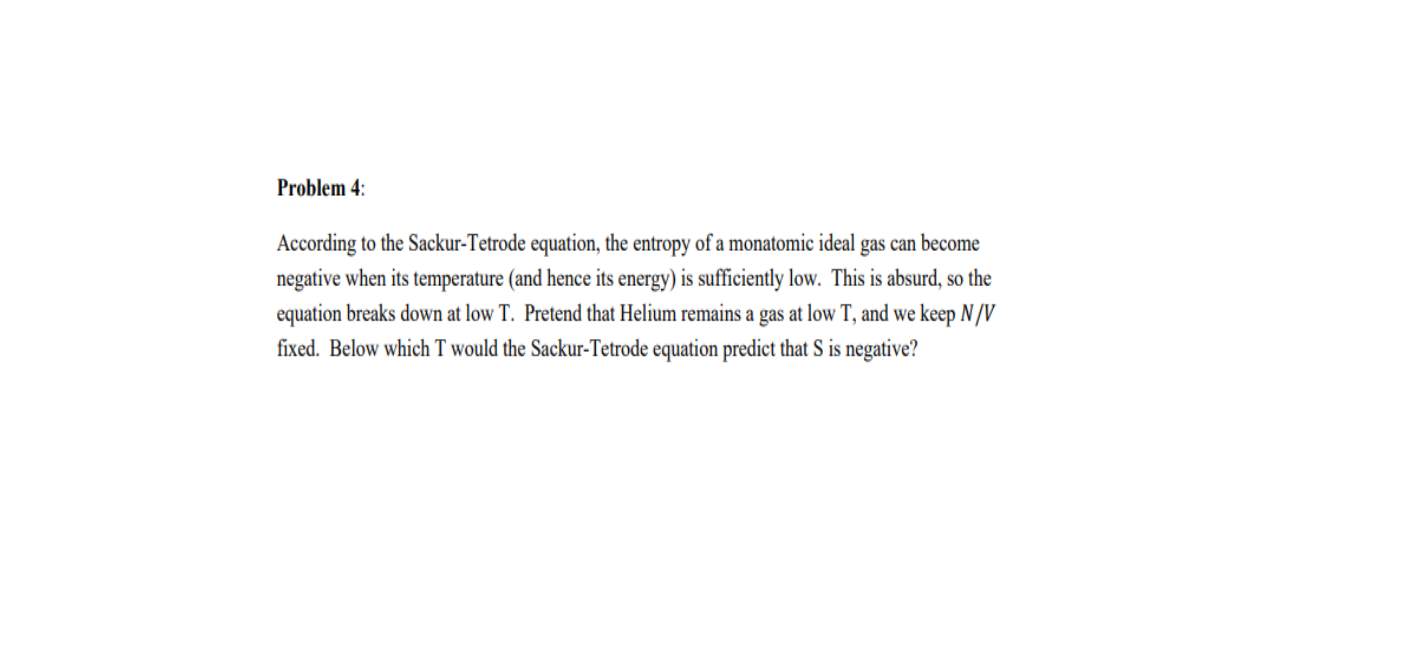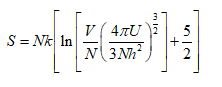# Problem 4:According to the Sackur-Tetrode equation, the entropy of a monatomic ideal gas can becomenegative when its temperature (and hence its energy) is sufficiently low. This is absurd, so theequation breaks down at low T. Pretend that Helium remains a gas at low T, and we keep N /Vfixed. Below which T would the Sackur-Tetrode equation predict that S is negative?

Question
37 viewshelp_outlineImage TranscriptioncloseProblem 4: According to the Sackur-Tetrode equation, the entropy of a monatomic ideal gas can become negative when its temperature (and hence its energy) is sufficiently low. This is absurd, so the equation breaks down at low T. Pretend that Helium remains a gas at low T, and we keep N /V fixed. Below which T would the Sackur-Tetrode equation predict that S is negative? fullscreen
check_circle

Sackur-Tetrode equation is given by,From the above expression,

It can be observed that when the internal energy U is low enough such that the log term goes below – 5/2, the entropy S becomes...

### Want to see the full answer?

See Solution

#### Want to see this answer and more?

Solutions are written by subject experts who are available 24/7. Questions are typically answered within 1 hour.*

See Solution
*Response times may vary by subject and question.
Tagged in